# Multiplicative comparison

Here you will learn about what multiplicative comparisons are and how to use them to solve word problems.

Students will first learn about multiplicative comparisons in 4th grade and expand that knowledge through 5th grade when working with comparison statements and in 6th grade when learning about ratios.

## What is multiplicative comparison?

A multiplicative comparison is a way of comparing two quantities by asking how many times larger or smaller one quantity is than the other quantity. Multiplicative comparisons involve the operations of multiplication and division to solve problems.

A model can help solve multiplicative comparison problems because it helps you to visualize the amounts that need to be compared and to find the unknown quantity.

Let’s look at a few examples.

Example 1:

Lucas and Ryan are working on a construction project for school. Lucas’s wood board is 5 times the length of Ryan’s board. If Ryan’s wooden board is 3 feet, how long is Lucas’s wooden board?

Draw a bar model to help visualize the situation.

Ryan has a 3 foot board.

From the model, you can see that Lucas’s board is 5 times the length of Ryan’s board. There are 5 groups of 3 feet.

So the equation is 5 \times 3=\text{ length of Lucas's board}

5 \times 3=15

Lucas’s board is 15 feet.

Example 2:

Mike has 3 lollipops. Michelle has 4 times as many lollipops as Mike. How many lollipops does Michelle have?

Draw a picture to model this situation.

Michelle has four times as many lollipops as Mike. So she has 4 groups of 3 lollipops.

The equation is 4 \times 3=\text { amount of lollipops}

4 \times 3=12

Michelle has 12 lollipops.

Example 3:

Jillian has 12 inches of hair ribbon. Suzanne has half that length. How long is Suzanne’s hair ribbon?

Draw a bar model.

The equation is, \cfrac{1}{2} \, \times 12=\text { length of Suzanne's ribbon}

OR

12 \div 2 = \text { length of Suzanne's ribbon}

12 \div 2=6

Suzanne’s ribbon is 6 inches long.

### What is multiplicative comparison?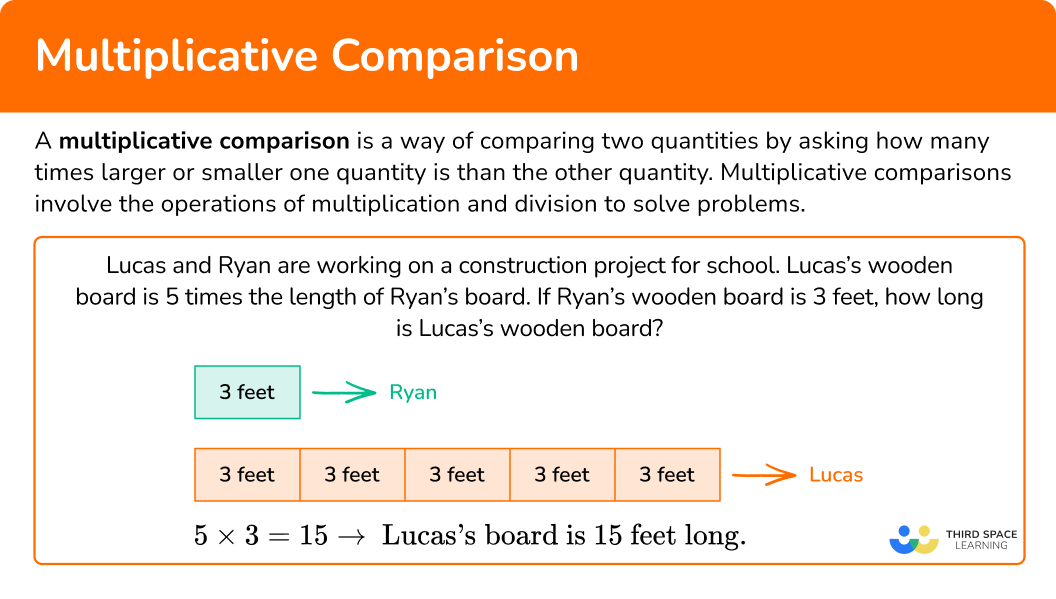## Common Core State Standards

How does this apply to 4th grade math and 5th grade math?

• Grade 4 – Operations and Algebraic Thinking (4.OA.A.1)
Interpret a multiplication equation as a comparison, for example, interpret 35 = 5 \times 7 as a statement that 35 is 5 times as many as 7 and 7 times as many as 5. Represent verbal statements of multiplicative comparisons as multiplication equations.

• Grade 4 – Operations and Algebraic Thinking (4.OA.A.2)
Multiply or divide to solve word problems involving multiplicative comparison, for example, by using drawings and equations with a symbol for the unknown number to represent the problem, distinguishing multiplicative comparison from additive comparison.

• Grade 5 – Number and Operations Base Ten – (5.NBT.B.7)
Add, subtract, multiply, and divide decimals to hundredths, using concrete models or drawings and strategies based on place value, properties of operations, and/or the relationship between addition and subtraction; relate the strategy to a written method and explain the reasoning used.

## How to solve multiplicative comparison problems

In order to solve multiplicative comparison problems:

1. Draw a model.
2. Use multiplication or division to write an equation.
3. Solve the equation.

## Multiplicative comparison examples

### Example 1: multiplicative comparison using a bar model with whole numbers

Maddie has collected 21 stickers. Her friend, Anna, has collected 3 times that amount. How many stickers does Anna have in her collection?

1. Draw a model.

Anna has 3 times the amount of stickers as Maddie. So she has 3 groups of 21.

2Use multiplication or division to write an equation.

The equation is 3 \times 21= \; ?

3 \times 21=63

Anna has 63 stickers.

### Example 2: multiplicative comparison using a bar model with whole numbers

Aderonke owns an animal shelter. She has 24 dogs in her shelter and twice as many cats. How many cats are in the shelter?

Draw a model.

Use multiplication or division to write an equation.

Solve the equation.

### Example 3: multiplicative comparison using a bar model

Amani has 18 yards of wire. Austin has a third of that length of wire. How much wire does Austin have?

Draw a model.

Use multiplication or division to write an equation.

Solve the equation.

Billy saved \$122 dollars. His sister, Nikki, has saved 4 times that amount. How much money has Nikki saved? Draw a model. Use multiplication or division to write an equation. Solve the equation. ### Example 5: multiplicative comparison with decimals Jerome’s neighbor has 92 yards of fencing. Jerome has a fourth of that amount of fencing. How much fencing does Jerome have? Draw a model. Use multiplication or division to write an equation. Solve the equation. ### Example 6: multiplicative comparison with fractions Carl has 5 \, \cfrac{1}{2} \, gallons of paint. Lucy has three times that amount. How many gallons of paint does Lucy have? Draw a model. Use multiplication or division to write an equation. Solve the equation. ### Teaching tips for multiplicative comparison • Connect visual models to the equations so students can see the visual representation of the abstract equation. • Math worksheets have their place in a math lesson, but providing students with alternative opportunities to practice such as math games or digital platforms are more engaging. • Another visual representation that can be used when doing multiplicative comparison word problems is the number line. • Incorporate projects such as having students create their own multiplicative comparison word problems with answer keys and share them on the Google Classroom. ### Easy mistakes to make • Confusing when to use multiplication versus when to use division For example, when you are given an amount such as 18 and are asked to find 3 times that amount, use multiplication. However, if you are given the amount of 18 and are asked to find a third of that amount, use division. ### Practice multiplicative comparison problems 1. Doug has 16 pieces of candy. Dhalia has 3 times that amount. How many pieces of candy does Dhalia have? 32 \text{ pieces of candy}48 \text{ pieces of candy}5 \text{ pieces of candy}38 \text{ pieces of candy}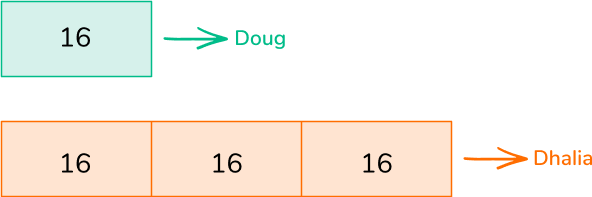Dhalia has 3 times the amount of candy as Doug which means she has 3 groups of 16. 3 \times 16=48 Dhalia has 48 pieces of candy. 2. Danni has 35 pens in her classroom. Jo has 5 times that amount. How many pens does Jo have in her classroom? 7 \text{ pens}170 \text{ pens}5 \text{ pens}175 \text{ pens}Jo has 5 times as many pens as Danni which means she has 5 groups of 35. 5\times 35=175 Jo has 175 pens in her classroom. 3. Coach Tony has 82 students trying out for his volleyball team. Coach Luke has half that amount trying out for his soccer team. Which equation represents the amount of students trying out for Coach Luke’s soccer team? 82 \times 2= \; ?82+2= \; ?82 \div 2= \; ?82 \div \cfrac{1}{2}= \; ?Coach Luke has half the number of students trying out for his soccer team than Coach Tony which means 82 is divided into 2 equal groups. 82 \div 2=41 4. Devin has \$243.30 in her savings account. Debra has a third of that amount in her savings account. How much money does Debra have?

\$729.30\$121.50\$486.30\$8.10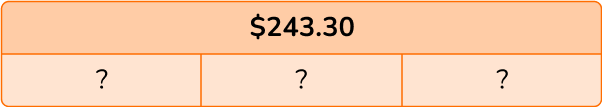Debra has a third of the amount of money as Devin. So \$243.30 has to be divided into 3 equal groups. 243.30 \div 3=81.10 Debra has \$81.10 .

5. Julie has 4 \, \cfrac{2}{3} \, feet of yarn. Dylan has 6 times that amount. How much yarn does Dylan have?

28 \text { feet of yarn}28 \, \cfrac{2}{3} \text { feet of yarn}28 \, \cfrac{1}{3} \text { feet of yarn}28 \, \cfrac{1}{2} \text { feet of yarn}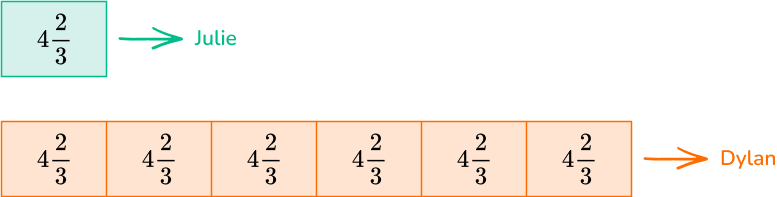Dylan has 6 times the amount of yarn as Julie, which means 6 groups of 4 \, \cfrac{2}{3} \, .

\begin{aligned} & 6 \times 4 \, \cfrac{2}{3} \, = \\\\ & 6 \times \cfrac{14}{3} \, = \cfrac{84}{3}=28 \end{aligned}

Dylan has 28 feet of yarn.

6. Rory planted a vegetable garden. He planted 51 tomato plants and a third the amount of zucchini plants. How many zucchini plants did he plant?

16 zucchini plants17 zucchini plants18 zucchini plants19 zucchini plants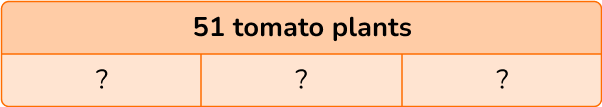Rory planted a third of the number of tomato plants which means 51 is divided into 3 equal groups.

51 \div 3=17

OR

\cfrac{1}{3} \, \times 51=17

## Multiplicative comparison FAQs

Do you always have to write an equation when solving a multiplicative comparison word problem?

No, you do not always have to write an equation. However, writing equations is a skill necessary for secondary mathematics.

## Still stuck?

At Third Space Learning, we specialize in helping teachers and school leaders to provide personalized math support for more of their students through high-quality, online one-on-one math tutoring delivered by subject experts.

Each week, our tutors support thousands of students who are at risk of not meeting their grade-level expectations, and help accelerate their progress and boost their confidence.